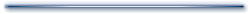# Calculators

Ohm's Law

Enter two of the values below and press compute:

 E= Voltage V mV µV This calculator computes Ohm's law. If you know any two values the calculator will find the other value. Hint: You can rescale the answer using the drop down boxes. I= Current in A mA µA R= Resistance in Ω kΩ MΩResonant Frequency

Enter two of the values below and press compute:

 F= Frequency in Hz kHz MHz This calculator computes the resonant frequency of a coil and capacitor. If you know any two values the calculator will find the other value. Hint: You can rescale the answer using the drop down boxes. L= Inductance in H mH µH nH C= Capacitance in F µF nF pFParallel Components

Enter values below and press compute:

 Value= Use one unit of entry This calculator computes the effective value of resistors or inductors in parallel. It also computes the value of capacitors in series. Use the same units for all entries (e.g., ohms, microfarads, etc.). Each click of the compute button will add another component. Use Clear to start over.